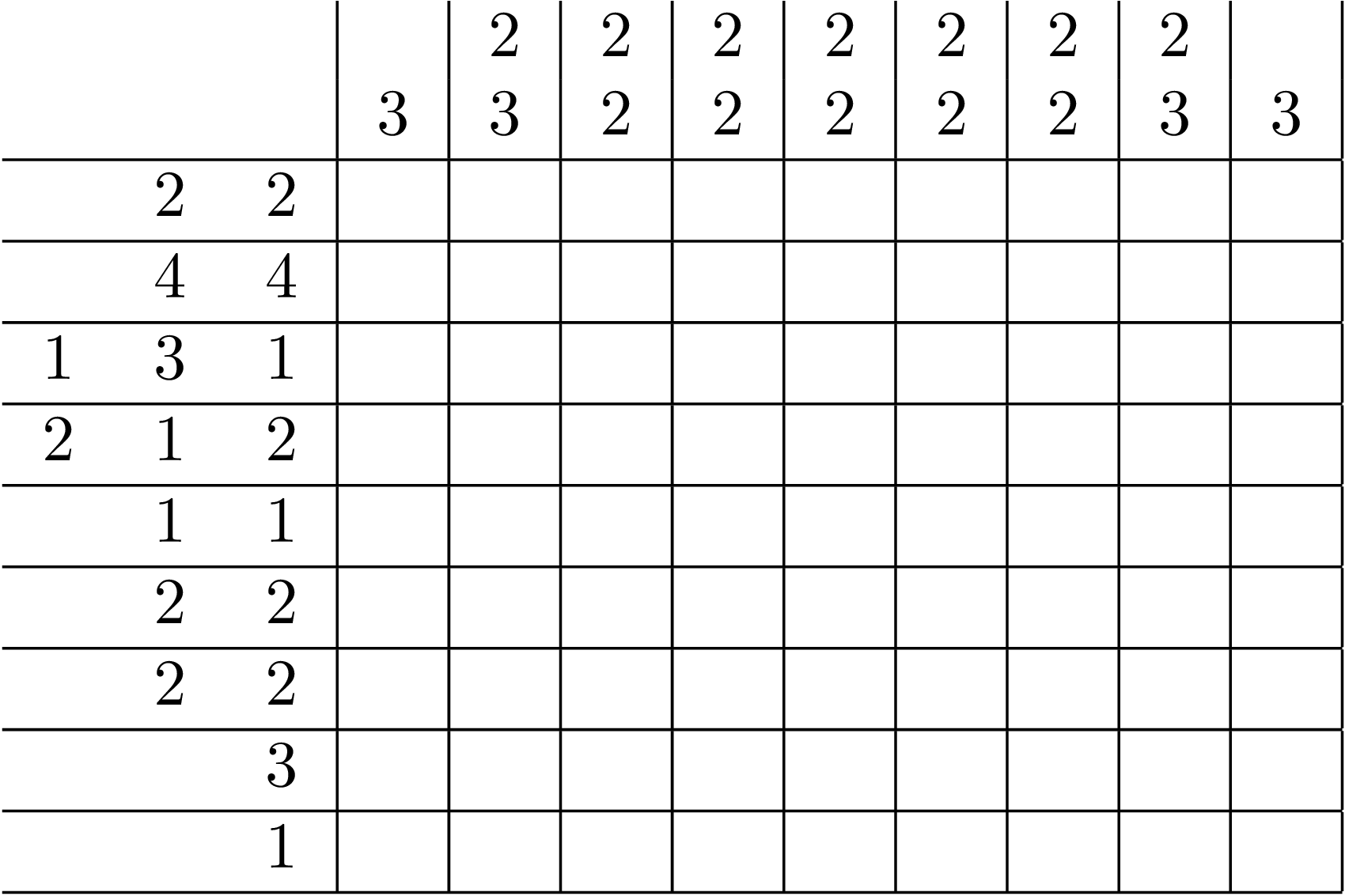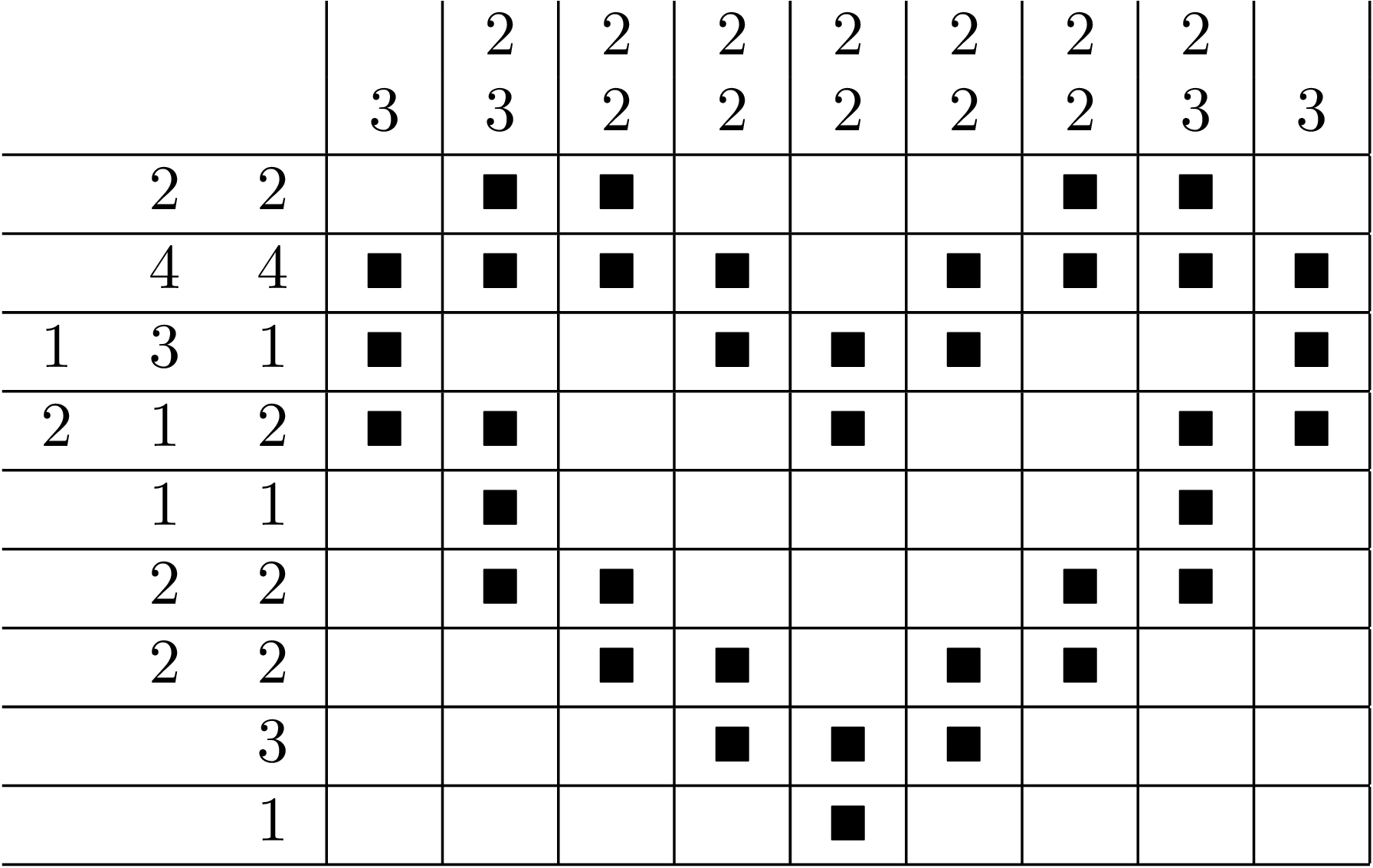PyCSP3

type: CSP
difficulty: medium

# Problem Nonogram

From CSPLib: Nonograms are a popular puzzle, which goes by different names in different countries. Solvers have to shade in squares in a grid so that blocks of consecutive shaded squares satisfy constraints given for each row and column. Constraints typically indicate the sequence of shaded blocks (e.g. 3,1,2 means that there is a block of 3, then a gap of unspecified size, a block of length 1, another gap, and then a block of length 2). Below, there is an example (taken from Chapter 14 in Gecode documentation):

Puzzle:Solution:To build a CSP (Constraint Satisfaction Problem) model, we need first to import the library PyCSP$^3$:

from pycsp3 import *


Suppose that the data (for a specific Nonogram puzzle) are initially given in a text file as follows:

• a line stating the numbers of rows and columns,
• then, for each row a line stating the number of blocks followed by the sizes of all these blocks (on the same line),
• then, for each column a line stating the number of blocks followed by the sizes of all these blocks (on the same line).
9 9
2  2 2
2  4 4
3  1 3 1
3  2 1 2
2  1 1
2  2 2
2  2 2
1  3
1  1

1  3
2  2 3
2  2 2
2  2 2
2  2 2
2  2 2
2  2 2
2  2 3
1  3


It is possible to loas such a file, for example with:

from pycsp3.problems.data.parsing import register_fields, next_int

register_fields("https://www.cril.univ-artois.fr/~lecoutre/heart.txt")

nRows, nCols = next_int(), next_int()
rows = [[next_int() for _ in range(next_int())] for _ in range(nRows)]
cols = [[next_int() for _ in range(next_int())] for _ in range(nRows)]


However, here, we will consider a JSON file. Actually, this file can be found here. To load block patterns, we can then execute:

rows, cols = default_data("https://www.cril.univ-artois.fr/~lecoutre/heart.json")
nRows, nCols = len(rows), len(cols)


We can check data:

print("Patterns for rows: ", rows)
print("Patterns for columns: ", cols)

Patterns for rows:  [
[2, 2]
[4, 4]
[1, 3, 1]
[2, 1, 2]
[1, 1]
[2, 2]
[2, 2]


]
Patterns for columns:  [

[2, 3]
[2, 2]
[2, 2]
[2, 2]
[2, 2]
[2, 2]
[2, 3]

]


We start our CSP model with a two-dimensional array $x$ of variables, each variable having {0,1} as domain.

# x[i][j] is 1 iff the cell at row i and col j is colored in black
x = VarArray(size=[nRows, nCols], dom={0, 1})


We can display (the structure of) the array as well as the domain of the first variable.

print("Array of variables x: ", x)
print("Domain of any variable: ", x.dom)

Array of variables x:  [
[x, x, x, x, x, x, x, x, x]
[x, x, x, x, x, x, x, x, x]
[x, x, x, x, x, x, x, x, x]
[x, x, x, x, x, x, x, x, x]
[x, x, x, x, x, x, x, x, x]
[x, x, x, x, x, x, x, x, x]
[x, x, x, x, x, x, x, x, x]
[x, x, x, x, x, x, x, x, x]
[x, x, x, x, x, x, x, x, x]
]
Domain of any variable:  0 1


We need to post a constraint Regular per row and per column. Indeed, one can transform any clue (pattern for a row or column) into an automaton. We use this function:

def automaton(pattern):
q = Automaton.q  # for building state names
transitions = []
if len(pattern) == 0:
n_states = 1
transitions.append((q(0), 0, q(0)))
else:
n_states = sum(pattern) + len(pattern)
num = 0
for i, size in enumerate(pattern):
transitions.append((q(num), 0, q(num)))
transitions.extend((q(num + j), 1, q(num + j + 1)) for j in range(size))
transitions.append((q(num + size), 0, q(num + size + (1 if i < len(pattern) - 1 else 0))))
num += size + 1
return Automaton(start=q(0), final=q(n_states - 1), transitions=transitions)



For example, if we display the automaton corresponding to the clue (2 2) given for the first row, we can check that the automaton is well built.

print(automaton(rows))

Automaton(start=q0, transitions={(q0,0,q0),(q0,1,q1),(q1,1,q2),(q2,0,q3),(q3,0,q3),(q3,1,q4),(q4,1,q5),(q5,0,q5)}, final=[q5])


We can now post the constraints Regular:

satisfy(
[x[i] in automaton(rows[i]) for i in range(nRows)],

[x[:,j] in automaton(cols[j]) for j in range(nCols)]
);


Interestingly, by calling the function solve(), we can check that the problem is satisfiable (SAT). We can also display the found solution. Here, we call the function values() that collects the values assigned to a specified list of variables.

if solve() is SAT:
print(values(x))

[
[0, 1, 1, 0, 0, 0, 1, 1, 0]
[1, 1, 1, 1, 0, 1, 1, 1, 1]
[1, 0, 0, 1, 1, 1, 0, 0, 1]
[1, 1, 0, 0, 1, 0, 0, 1, 1]
[0, 1, 0, 0, 0, 0, 0, 1, 0]
[0, 1, 1, 0, 0, 0, 1, 1, 0]
[0, 0, 1, 1, 0, 1, 1, 0, 0]
[0, 0, 0, 1, 1, 1, 0, 0, 0]
[0, 0, 0, 0, 1, 0, 0, 0, 0]
]


We can improve the output:

if solve() is SAT:
for i in range(nRows):
print(' '.join('*' if value(x[i][j]) == 1 else ' ' for j in range(nCols)))

  * *       * *
* * * *   * * * *
*     * * *     *
* *     *     * *
*           *
* *       * *
* *   * *
* * *
*


Finally, we give below the model in one piece. Here the data is expected to be given by the user (in a command line).

from pycsp3 import *

rows, cols = data  # patterns for row and columns
nRows, nCols = len(rows), len(cols)

# x[i][j] is 1 iff the cell at row i and col j is colored in black
x = VarArray(size=[nRows, nCols], dom={0, 1})

def automaton(pattern):
q = Automaton.q  # for building state names
transitions = []
if len(pattern) == 0:
n_states = 1
transitions.append((q(0), 0, q(0)))
else:
n_states = sum(pattern) + len(pattern)
num = 0
for i, size in enumerate(pattern):
transitions.append((q(num), 0, q(num)))
transitions.extend((q(num + j), 1, q(num + j + 1)) for j in range(size))
transitions.append((q(num + size), 0, q(num + size + (1 if i < len(pattern) - 1 else 0))))
num += size + 1
return Automaton(start=q(0), final=q(n_states - 1), transitions=transitions)

satisfy(
[x[i] in automaton(rows[i]) for i in range(nRows)],

[x[:, j] in automaton(cols[j]) for j in range(nCols)]
)